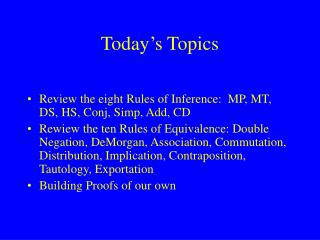DownloadDownload PresentationToday’s Topics

# Today’s Topics

Download Presentation## Today’s Topics

- - - - - - - - - - - - - - - - - - - - - - - - - - - E N D - - - - - - - - - - - - - - - - - - - - - - - - - - -
##### Presentation Transcript

1. Today’s Topics • Review the eight Rules of Inference: MP, MT, DS, HS, Conj, Simp, Add, CD • Rewiew the ten Rules of Equivalence: Double Negation, DeMorgan, Association, Commutation, Distribution, Implication, Contraposition, Tautology, Exportation • Building Proofs of our own

2. Modus Ponens (MP) From a conditional and a line identical to its antecedent, you may derive a line identical to its consequent Modus Tollens (MT) From a conditional and the negations of its consequent, you may derive the negation of its antecedent Disjunctive Syllogism (DS) From a disjunction and the negation of one disjunct, you may derive the other disjunct Hypothetical Syllogism (HS) From 2 conditionals, if the consequent of the first is identical to the antecedent of the second, you may derive a new conditonal whose antecedent is identical to the antecedent of the first and whose consequent is identical to the consequent of the second. Eight Basic Inference Rules

3. Modus Ponens Modus Tollens p  q p  q p ~q q~p Disjunctive Syllogism Hypothetical Syllogism p  q p  q ~p q  r qp r

4. Simplification From a conjunction you may derive either conjunct. Conjunction From any 2 lines you may derive a conjunction which has those lines as conjuncts Addition From any line you may derive a disjunction with that line as a disjunct Constructive Dilemma From a disjunction and 2 conditionals, if the antecedents match the disjuncts, you may derive a disjunction of the consequents

5. Simplification p  q p q Conjunction p q p  q Addition p p v q Constructive Dilemma p v q p  r q  s r v s

6. Double Negation (DN) p :: ~ ~ p DeMorgan (DM) ~(p v q) :: (~p  ~q) ~(p  q) :: (~p v ~q) Association (Assn) (p v q) v r :: p v (q v r) (p  q)  r :: p  (q  r) Commutation (Comm) p v q :: q v p p  q :: q  p Rules of Equivalence

7. Distribution (Dist) [p  (q v r)] :: [(p  q) v (p  r)] [p v (q  r)] :: [(p v q)  (p v r)] Implication (Impl) (p  q) :: (~p v q) Exportation (Exp) ((p q)  r) :: (p  (q  r))

8. Contraposition (Contra) (p  q) :: (~q  ~p) Tautology (Taut) p :: (p  p) p :: (p v p) Equivalence (Equiv) (p  q) :: [(p  q) v (~p ~q)] (p  q) :: [(p  q)  (q  p)]

9. Exercises • 4-5 problems 1-20